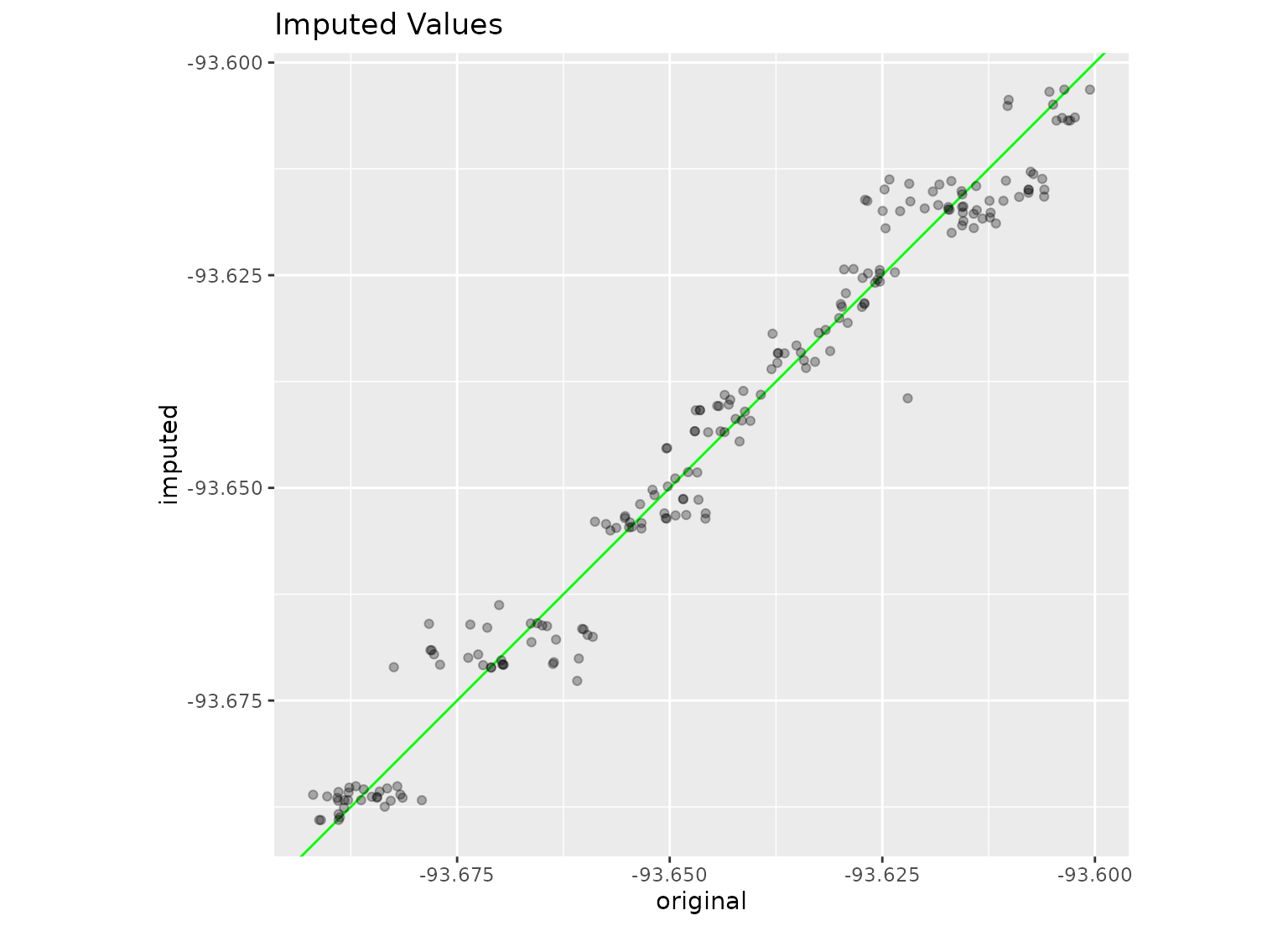step_impute_linear creates a specification of a recipe step that will create linear regression models to impute missing data.

step_impute_linear(
recipe,
...,
role = NA,
trained = FALSE,
impute_with = imp_vars(all_predictors()),
models = NULL,
skip = FALSE,
id = rand_id("impute_linear")
)

## Arguments

recipe A recipe object. The step will be added to the sequence of operations for this recipe. One or more selector functions to choose variables to be imputed; these variables must be of type numeric. When used with imp_vars, these dots indicate which variables are used to predict the missing data in each variable. See selections() for more details. Not used by this step since no new variables are created. A logical to indicate if the quantities for preprocessing have been estimated. A call to imp_vars to specify which variables are used to impute the variables that can include specific variable names separated by commas or different selectors (see selections()). If a column is included in both lists to be imputed and to be an imputation predictor, it will be removed from the latter and not used to impute itself. The lm() objects are stored here once the linear models have been trained by prep.recipe(). A logical. Should the step be skipped when the recipe is baked by bake.recipe()? While all operations are baked when prep.recipe() is run, some operations may not be able to be conducted on new data (e.g. processing the outcome variable(s)). Care should be taken when using skip = TRUE as it may affect the computations for subsequent operations. A character string that is unique to this step to identify it.

## Value

An updated version of recipe with the new step added to the sequence of any existing operations.

## Details

For each variable requiring imputation, a linear model is fit where the outcome is the variable of interest and the predictors are any other variables listed in the impute_with formula. Note that if a variable that is to be imputed is also in impute_with, this variable will be ignored.

The variable(s) to be imputed must be of type numeric. The imputed values will keep the same type as their original data (i.e, model predictions are coerced to integer as needed).

Since this is a linear regression, the imputation model only uses complete cases for the training set predictors.

When you tidy() this step, a tibble with columns terms (the selectors or variables selected) and model (the bagged tree object) is returned.

Kuhn, M. and Johnson, K. (2013). Feature Engineering and Selection https://bookdown.org/max/FES/handling-missing-data.html

Other imputation steps: step_impute_bag(), step_impute_knn(), step_impute_lower(), step_impute_mean(), step_impute_median(), step_impute_mode(), step_impute_roll()

## Examples

data(ames, package = "modeldata")
set.seed(393)
ames_missing <- ames
ames_missing\$Longitude[sample(1:nrow(ames), 200)] <- NA

imputed_ames <-
recipe(Sale_Price ~ ., data = ames_missing) %>%
step_impute_linear(
Longitude,
impute_with = imp_vars(Latitude, Neighborhood, MS_Zoning, Alley)
) %>%
prep(ames_missing)

imputed <-
bake(imputed_ames, new_data = ames_missing) %>%
dplyr::rename(imputed = Longitude) %>%
bind_cols(ames %>% dplyr::select(original = Longitude)) %>%
bind_cols(ames_missing %>% dplyr::select(Longitude)) %>%
dplyr::filter(is.na(Longitude))

library(ggplot2)
ggplot(imputed, aes(x = original, y = imputed)) +
geom_abline(col = "green") +
geom_point(alpha = .3) +
coord_equal() +
labs(title = "Imputed Values")